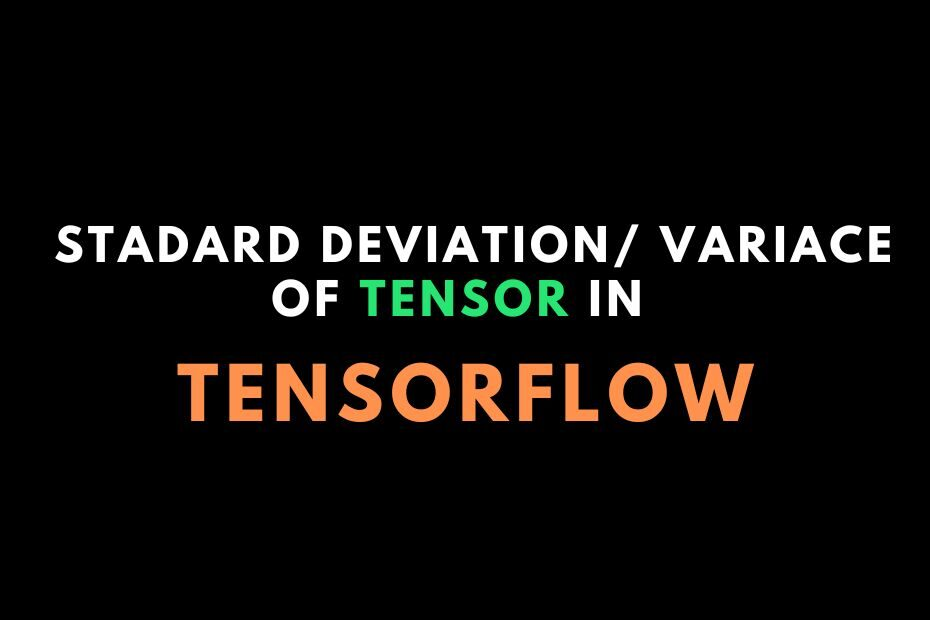# Easy way to calculate the standard deviation or variance of the tensor in TensorFlow?Let’s see how you can calculate the standard deviation or variance of the tensor. As you know while building a neural network, you have to perform aggregation on the tensor often. Finding maximum, minimum, and sum is done via the same piece of code with very little change in it. But finding variance or standard deviation will require some tweaks.

## Calculate the Minimum, Maximum, and Sum of the Tensor

First of all, let’s see how you can get the maximum, minimum, and sum of the tensor if you don’t know.

You can find the maximum, minimum, and sum of the tensor by writing `tf.reduce_max()`,`tf.reduce_min()`, and `tf.reduce_sum()` accordingly.

Let’s see all of these in python. Here I have converted to stored value to a numpy array in the print statement. Because the `tf.reduce` method gives a tensor object as an output.

```import tensorflow as tf

A=tf.constant([[10,11,12],[13,14,15]])

max_A=tf.reduce_max(A)

min_A=tf.reduce_min(A)

sum_A=tf.reduce_sum(A)

print("The Maximum of Tensor A: ",max_A.numpy())
print("The Minimum of Tensor A: ",min_A.numpy())
print("The Sum of Tensor A: ",sum_A.numpy())
```

Output:

```The Maximum of Tensor A:  15
The Minimum of Tensor A:  10
The Sum of Tensor A:  75
```

## Calculate the standard deviation or variance of the tensor in TensorFlow

Finding Variance or Standard deviation has the same procedure as you did for finding minimum, maximum, and sum.

But here is the twist. First is that you have to import it from `tf.math` module. And second, If you have an integer type of value in the tensor, then it will give an error if you pass integer values in it. Because it only accepts real or complex values.

And in real values, it accepts floa32 or float16 type of values. So if you must have to convert integer(if you have) type values to the floating type. You can cast the type of values by calling `tf.cast()` method.

Here I have created 50 random numbers from the range of 0 to 100. Let’s find out the probability of this:

```import tensorflow as tf
import numpy as np

E=tf.constant(np.random.randint(0,100,size=50))

print(E)

var_E=tf.math.reduce_variance(tf.cast(E,dtype=tf.float32))

print("--The Variance of E is:", var_E.numpy())

std_E=tf.math.reduce_std(tf.cast(E,dtype=tf.float32))

print("--The Stadard deviation of E is:", std_E.numpy())
```

Output:

```tf.Tensor(
[52 10 34 24 89 70 60  0 77 66 23 94 65 14 26 51 71 44 75 53 15 23 29 72
39 76 48 31 98 72 49 77 56 51 57 79 11 76 50 90 93 29 39 43 65 81  1 75
57 63], shape=(50,), dtype=int64)
--The Variance of E is: 653.6004
--The Stadard deviation of E is: 25.565609
```

Alternative Solution: You can get the standard deviation or Variance of the tensor by using the Tensorflow Probability library(tfp).

So that’s how you can calculate the standard deviation or variance of the tensor.

If you are facing any difficulties in these, let me know in the comments.